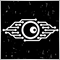Português

# error 'char' - unexpected token ; All Stochastic v1.0.mq430

hello guys I have an MT4 indicator, but this is giving me the following error in the source code, as I'm not a programmer I don't understand any of this, the indicator and the All Stochastic mq4.

this and the entire code of the indicator, if anyone knows how to solve these error messages, could you help me?

```//+------------------------------------------------------------------+
//|                                               All Stochastic.mq4 |
//+------------------------------------------------------------------+
#property copyright   "this is public domain software"
#define indicatorName "All Stochastic"

//
//
//
//
//

#property indicator_separate_window
#property indicator_minimum 0
#property indicator_maximum 100
#property indicator_buffers 2
#property indicator_color1  Gold
#property indicator_color2  Red
#property indicator_style2  STYLE_DOT
#property indicator_level1  20
#property indicator_level3  80
#property indicator_levelcolor DimGray

//---- input parameters
//
//
//
//
//

extern int    Kperiod              = 5;
extern int    Dperiod              = 3;
extern int    Slowing              = 3;
extern int    MAMethod             = 0;
extern int    PriceField           = 0;
extern string __                   = "Chose timeframes (as in periodicity bar)";
extern string timeFrames           = "M1;M5;M15;M30;H1;H4;D1;W1;MN";
extern int    barsPerTimeFrame     = 35;
extern bool   shiftRight           = False;
extern bool   currentFirst         = False;
extern color  txtColor             = Silver;
extern color  separatorColor       = DimGray;

//---- buffers
//
//
//
//
//

double ExtMapBuffer1[];
double ExtMapBuffer2[];

//
//
//
//
//

string shortName;
string labels[];
int    periods[];
int    Shift;

//+------------------------------------------------------------------+
//| Custom indicator initialization function                         |
//+------------------------------------------------------------------+

int init()
{
if (shiftRight) Shift = 1;
else            Shift = 0;
barsPerTimeFrame = MathMax(barsPerTimeFrame,15);
shortName = indicatorName+" ("+Kperiod+","+Dperiod+","+Slowing+")";
IndicatorShortName(shortName);

//
//
//
//
//

SetIndexBuffer(0,ExtMapBuffer1);
SetIndexBuffer(1,ExtMapBuffer2);

SetIndexShift(0,Shift*(barsPerTimeFrame+1));
SetIndexShift(1,Shift*(barsPerTimeFrame+1));
SetIndexLabel(0,"Stochastic");
SetIndexLabel(1,"Signal");

//
//
//
//
//

timeFrames = StringUpperCase(StringTrimLeft(StringTrimRight(timeFrames)));
if (StringSubstr(timeFrames,StringLen(timeFrames),1) != ";")
timeFrames = StringConcatenate(timeFrames,";");

//
//
//
//
//

int s = 0;
int i = StringFind(timeFrames,";",s);
int time;
string current;
while (i > 0)
{
current = StringSubstr(timeFrames,s,i-s);
time    = stringToTimeFrame(current);
if (time > 0) {
ArrayResize(labels ,ArraySize(labels)+1);
ArrayResize(periods,ArraySize(periods)+1);
labels[ArraySize(labels)-1] = current;
periods[ArraySize(periods)-1] = time; }
s = i + 1;
i = StringFind(timeFrames,";",s);
}

//
//
//
//
//

if(currentFirst)
for (i=1;i<ArraySize(periods);i++)
if (Period()==periods[i])
{
string tmpLbl = labels[i];
int    tmpPer = periods[i];

//
//
//
//
//

for (int k=i ;k>0; k--) {
labels[k]  = labels[k-1];
periods[k] = periods[k-1];
}
labels  = tmpLbl;
periods = tmpPer;
}
return(0);
}

//+------------------------------------------------------------------+
//| Custom indicator deinitialization function                       |
//+------------------------------------------------------------------+
int deinit()
{
for(int l=0;l<ArraySize(periods);l++) {
ObjectDelete(indicatorName+l);
ObjectDelete(indicatorName+l+"label");
}
return(0);
}

//+------------------------------------------------------------------+
//| Custom indicator iteration function                              |
//+------------------------------------------------------------------+

int start()
{
string separator;
int    window=WindowFind(shortName);
int    k=0;

//
//
//
//
//

for(int p=0; p<ArraySize(periods);p++)
{
for(int i=0; i<barsPerTimeFrame;i++,k++)
{
ExtMapBuffer1[k] = iStochastic(NULL,periods[p],Kperiod,Dperiod,Slowing,MAMethod,PriceField,0,i);
ExtMapBuffer2[k] = iStochastic(NULL,periods[p],Kperiod,Dperiod,Slowing,MAMethod,PriceField,1,i);
}
ExtMapBuffer1[k] =EMPTY_VALUE;
ExtMapBuffer2[k] =EMPTY_VALUE;
k += 1;

//
//
//
//
//

separator = indicatorName+p;
if(ObjectFind(separator)==-1)
ObjectCreate(separator,OBJ_TREND,window,0,0);
ObjectSet(separator,OBJPROP_TIME1,barTime(k-Shift*(barsPerTimeFrame+1)-1));
ObjectSet(separator,OBJPROP_TIME2,barTime(k-Shift*(barsPerTimeFrame+1)-1));
ObjectSet(separator,OBJPROP_PRICE1,  0);
ObjectSet(separator,OBJPROP_PRICE2,100);
ObjectSet(separator,OBJPROP_COLOR ,separatorColor);
ObjectSet(separator,OBJPROP_WIDTH ,2);
separator = indicatorName+p+"label";
if(ObjectFind(separator)==-1)
ObjectCreate(separator,OBJ_TEXT,window,0,0);
ObjectSet(separator,OBJPROP_TIME1,barTime(k-Shift*(barsPerTimeFrame+1)-5));
ObjectSet(separator,OBJPROP_PRICE1,100);
ObjectSetText(separator,labels[p],9,"Arial",txtColor);
}

//
//
//
//
//

SetIndexDrawBegin(0,Bars-k);
SetIndexDrawBegin(1,Bars-k);
return(0);
}

//+------------------------------------------------------------------+
//+ Custom functions and procedures                                  +
//+------------------------------------------------------------------+

int barTime(int a)
{
if(a<0)
return(Time+Period()*60*MathAbs(a));
else  return(Time[a]);
}

//+------------------------------------------------------------------+
//+                                                                  +
//+------------------------------------------------------------------+
//
//
//
//
//

int stringToTimeFrame(string TimeFrame)
{
int TimeFrameInt=0;
if (TimeFrame=="M1")  TimeFrameInt=PERIOD_M1;
if (TimeFrame=="M5")  TimeFrameInt=PERIOD_M5;
if (TimeFrame=="M15") TimeFrameInt=PERIOD_M15;
if (TimeFrame=="M30") TimeFrameInt=PERIOD_M30;
if (TimeFrame=="H1")  TimeFrameInt=PERIOD_H1;
if (TimeFrame=="H4")  TimeFrameInt=PERIOD_H4;
if (TimeFrame=="D1")  TimeFrameInt=PERIOD_D1;
if (TimeFrame=="W1")  TimeFrameInt=PERIOD_W1;
if (TimeFrame=="MN")  TimeFrameInt=PERIOD_MN1;
return(TimeFrameInt);
}

//
//
//
//
//

string StringUpperCase(string str)
{
string   s = str;
int      lenght = StringLen(str) - 1;
int      char;

while(lenght >= 0)
{
char = StringGetChar(s, lenght);

//
//
//
//
//

if((char > 96 && char < 123) || (char > 223 && char < 256))
s = StringSetChar(s, lenght, char - 32);
else
if(char > -33 && char < 0)
s = StringSetChar(s, lenght, char + 224);

//
//
//
//
//

lenght--;
}

//
//
//
//
//

return(s);
}```131330

32139125:

hello guys I have an MT4 indicator, but this is giving me the following error in the source code, as I'm not a programmer I don't understand any of this, the indicator and the All Stochastic mq4.

this and the entire code of the indicator, if anyone knows how to solve these error messages, could you help me?

Você está compilando este código em qual Metatrader?  4 ou 5?

E, estamos em um fórum em português, portanto, escreva em português...30

mt4, não sabeia que era em português.